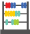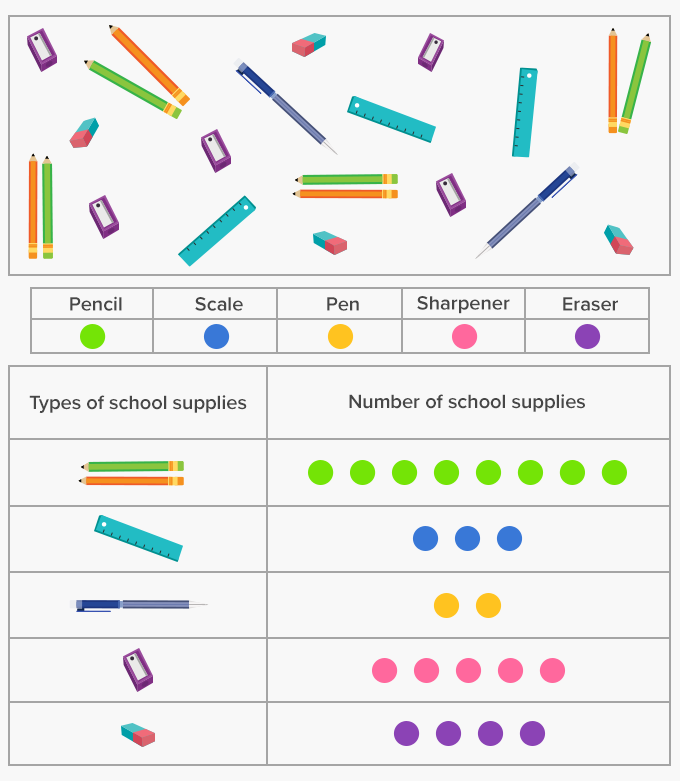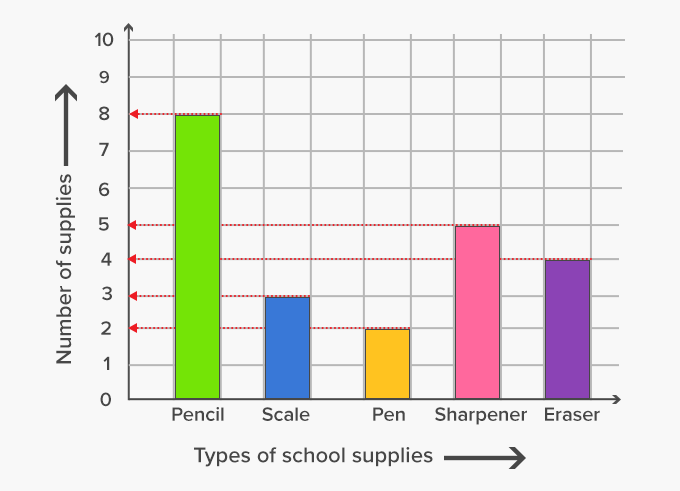# Graph - Definition with Examples

The Complete Prek-5 Learning Program Built for Your Child
Home > Graph

##Let's learn!

What is a graph?
In math, a graph can be defined as a pictorial representation or a diagram that represents data or values in an organized manner.

The points on the graph often represent the relationship between two or more things.

Here, for instance, we can represent the data given below, the type and number of school supplies used by students in a class, on a graph. We begin by counting each supply and representing the data in particular colors in a systematic order in a table.We then represent the data using a bar graph. The number of each of the supplies is represented with bars. The more the height of the bar, the more is the number of the supply or item used.Fun Facts There are different types of graphs. Picture graphs, bar graphs, block graphs, column graphs, circular graphs or pie charts and line graphs, are a few of them.

##Let's sing!

The number of lions, the number of its giraffes.
The number of men and women in a school staff,
The number of words written in each paragraph,
All this data can be represented in math on a graph!

##Let's do it!

Instead of handing out worksheets or playing games around coloring bar graphs, give your child a chart paper and supplies such as a ruler, pencil and crayons. Ask them to represent the average population of 7 countries or the average number of 6-7 of their favorite animals in the world. Help them in sourcing the statistics. Ask them to mark the title, axis, scale and label the graph well. Also, help them in deciding the scale of the graph.

##Related math vocabulary